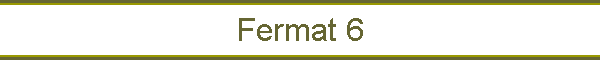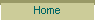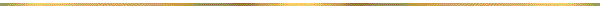"Dr. John Cosgrave's talk on number theory was for many people the best thing they've been to all year. He gave a wonderfully lucid account of his recent discoveries and ideas about Fermat numbers - accessable to all & extremely interesting for those of us (i.e. everyone) who hasn't actually seen a number theorist at work. The talk [which was about the Fermat 6 work on this page] actually managed to stretch on for more than two (very short & extremely enjoyable) hours, and it was well after twelve [midnight] before we let him leave the maths department." (Quoted from the Trinity College Dublin (Student) Mathematical Society web site. Thank you to whoever wrote the above; no bribes were offered!)Note of April 2021 I am currently trying to improve this page, after a gap of more than twenty years... What is this page about? It's about my response the (unanswered) question: How many Fermat primes are there? This page contains material relating to an e-mail which I sent to the Number Theory mailing list on 5th October 1999, following a question posted by Mingzhi Zhang on 4th October 1999 re primes of the form (2k + 2i + 1). Following this I submitted a paper (now, in Sept 2006, available in pdf format: Fermat_6), 'Could there exist a sixth Fermat prime? I believe it is not impossible', to the American Mathematical Monthly on 19th March 1999, and it was rejected. I have been asked why did I not submit it elsewhere, and the simple answer is that I lacked the emotional energy to do so; my paper made a certain obvious and elementary point, which some open minded people listened to respectfully, while others didn't. The e-mail of 20th March 1999 in which I made an announcement to a few friends and colleagues about my (new, I then thought; see later) unification of Fermat and Mersenne numbers : From: John B. Cosgrave To:          ... Subject: a unification of Mersenne and Fermat numbers Date: 20 March 1999 22:36 Dear Colleagues (and, in some cases, friends), I am writing to tell you of some work I have done in recent weeks, which, in a way, unifies into a whole the Fermat and Mersenne numbers. This work may (actually, should) throw doubt on the frequent assertion that the only Fermat primes are the first five (3, 5, 17, 257 and 65537). That was  not, of course, what I set out to do; rather I was just making some routine preparations for my third year Number Theory and Cryptography course (in connection with improving the statement of Proth's theorem, and its proof, so as to make it more accessible to my students), when a number of things took a turn ... (the actual details are in a paper that I have just completed, and submitted. Added later: it's the Fermat_6 paper above). Here, then, is my discovered linking of the Mersenne and Fermat numbers (if it is already buried out there in the literature, then obviously I apologise for wasting your time in reading this, and I end up with egg on face. Anyway, at the age of 53 I am not worried anymore about making a fool of myself): The Mersenne numbers are the Mp = 2p - 1, p prime; the Fermat numbers are the Fn = 22n + 1, n = 0, 1, 2, 3, 4, 5, ... . Currently there are 37 Mersenne primes (the last three found by George Woltman's wonderful GIMPS project, and there are only 5 known Fermat primes (3, 5, 17, 257 and 65537, from n = 0, 1, 2, 3, 4). I propose (as a pictorial image) that instead of thinking of those two sequences horizontally (as it were), one should see the Fermat numbers as being the vertical side, and the Mersenne numbers the horizontal side of this doubly infinite matrix of numbers: . . . ('level' 3)  F3, 1,     F3, 2,      F3, 3,     F3, 4, ... ('level' 2)  F2, 1,     F2, 2,      F2, 3,     F2, 4, ... ('level' 1)  F1, 1,     F1, 2,      F1, 3,     F1, 4, ... ('level' 0)  F0, 1,     F0, 2,      F0, 3,     F0, 4, ...          ('rank' 1)  ('rank' 2)  ('rank' 3)   ('rank' 4) ... where the left vertical side is comprised of the Fermat numbers (Fn,1 := Fn, n = 0, 1, 2, 3, 4 ... ), the bottom horizontal side is comprised of the Mersenne numbers F0, r := Mp, p the rth prime), and the general entry Fn, r is given by the value of the cyclotomic polynomial (xp-1 + xp-2 + ... + x + 1), evaluated at x = 2pn. Thus the 'ranks' are comprised of the numbers: rank 1: 22n + 1 (n = 0, 1, 2, 3, 4, ... ), the Fermat numbers, rank 2: 22.3n + 23n + 1 (n = 0, 1, 2, ... ), rank 3: 24.5n + 23.5n + 22.5n + 25n + 1 (n = 0, 1, 2, ... ), (n = 0, 1, 2, ... ), ... The numbers at level 0 are the numbers {F0, r}, with F0, r = 2p - 1 + 2p - 2 + ... + 2 + 1 = 2p - 1, and thus are the Mersenne numbers. The numbers at level 1 (I like to think of them as being the first cousins of the Mersenne numbers) are given by F1, r = 2p.(p-1) + 2p.(p-2) + 2p.(p-3) + ... + 2p + 1 = (2p2 - 1) / (2p - 1) These {Fn, r} are the (proposed) generalised Fermat numbers; (though it might be better to call them the Mersenne-Fermat numbers?), and are quite different from the already named generalised Fermat number (as in, e.g., Hans Riesel’s Prime Numbers and Computer Methods of Factorization), namely the Fn(a, b) = a2n + b2n, with gcd(a, b) = 1, and a, b of opposite parity. The proposed Fn, r have the following (easily proved) properties: 1. They satisfy a functional equation (similar to the classic Fermat F0 . F1 . F1. ... . . Fn-1 + 2 = Fn). ≠ 2. They are pairwise relatively prime (within and across the ranks, meaning: gcd(Fn, i, Fn, j) = 1, for i ≠ j, and gcd(Fm, i, Fn, j) = 1, for m ≠ n), 3. (the most interesting/significant one, in view of the fact that the Fermat numbers - as known - all pass the Lucas-Fermat test to the base 2, and indeed the same was known of the Mersenne numbers). Every Fn, r passes the Lucas-Fermat test to the base 2. And here, now, is how some considerable doubt must be cast over the belief/assertion that the Fermat numbers are all composite after the 5th of them. That belief may be equivalently restated as follows: a Fermat composite CANNOT be followed by a Fermat prime (meaning: if Fn is composite then Fn+1 CANNOT be prime) But the Fermat numbers are simply the left vertical side of the above doubly-infinite square, and what about the next rank along from it?, and the one alongside it?, and ... . Those numbers (in each rank) are like the Fermat numbers (the only difference is that they grow - of course - so much more quickly in size: F5 = F5, 1 = 225 +1 = 232 + 1, has 10 digits, F5, 2 has 147 digits, F5, 3 has 3763 digits, F5, 4 has 30357 digits, F5, 5 has 484812 digits, ... ). Now, my main point: Will anyone who holds to the common orthodoxy that there is no Fermat prime after the fifth (reminder: equivalent to the above a Fermat composite CANNOT be followed by a Fermat prime), will such a person not also maintain - by simple analogy - that for each of those ranks a prime (in that vertical rank) may not follow a composite in that same rank? Meaning if Fn, r is composite, isn't Fn, r+1 also composite? But I have found (there is a caveat, below) that at the 17th rank, where F0, 17 is M59, and, as is known M59 = 259 - 1, is composite, and is followed by the 1031 digit prime (see email from F. Morain below) F1, 17. I have not been able to prove that F1, 17 is prime by a classical method (say Pocklington, or Selfridge’s ‘change of base theorem’), and those of you who are familiar with such methods will immediately see why (I have explained this point in my paper). Maple (which I use) declares F1, 17 to be prime, but as you will know that cannot be relied upon for a number of 1031 digits. F1, 17 passes the Lucas-Fermat test to all prime bases up to 137 (I had to stop somewhere), and passes Miller's test for the first 25 primes (again I had to stop somewhere), and so is almost certainly prime (if not it will be the first counterexample to the Maple test; but no consolation!!) I have pointed all of this out to the Editor of the journal to which I have submitted my paper, and will (if he is agreeable) submit a request about proving (or disproving) primality to the Number Theory List. In the meantime I would ask you to regard all of the above as being a private communication.         I hope it has been of some interest to you.         With best wishes,         John 1.    An e-mail from Francois Morain: From: Francois Morain To: John Cosgrave Subject: Re: a unification of Mersenne and Fermat numbers Date: 25 March 1999 17:04 F[1,17] is prime. It took less than two days on a DecAlpha 500 MHz (V5). The certificate is available on request. F. Morain 2.   A later e-mail from me: From: John B. Cosgrave Subject: a conjectured primality test for Mersenne primes Date: 13 April 1999 08:38 Dear Colleagues/Friends, I am writing to all of you since many of you commented in some way on my recent circular note re a unification of Mersenne and Fermat numbers. I am now circulating the following, just discovered (rediscovered? - please inform me if you think so, and I will just give up), conjectured primality test for Mersenne primes: Let p be an odd prime, then Mp = 2p - 1 is prime if and only if 3((Mp - 1)/p) = 2a (mod Mp), for some integer a in the interval [0, p - 1]. One way is trivial, namely: if Mp is prime then 3((Mp - 1)/p) = 2a (mod Mp), a in the interval [0, p - 1], and I will not offend readers with a proof. I tested the converse for primes up to 50,000 and the above conjecture held good (but, as Daniel Shanks might well remark: 50,000 is a long way from infinity). What makes me a little hesitant about the above is the part played by '3', because, if one replaces '3' with (non-powers of 2, for obvious reasons) 5, 6, 7, 9, 10, 11, ... (to where?), then the corresponding test also seems to hold good. With best wishes, John Finally, I received some friendly communications in connection with this, but eventually a rejection of my paper by the Monthly.Contact details. jbcosgrave at gmail.com